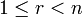# Conjugation family

## Definition

Let$G$ be a finite group and$P$ be a$p$-Sylow subgroup of$G$, for some prime$p$. A conjugation family for$S$ in$G$ is a family$\mathcal{F}$ of subgroups of$P$, such that the following holds.

### In terms of right actions

Whenever$A$ and$B$ are subsets of$P$ such that$A^g = B$ for some$g \in G$:

Then,$A$ and$B$ are$\mathcal{F}$-conjugate via$g$. In other words, we can find elements$g_1,g_2,\ldots,g_n \in G$, and subgroups$T_1,T_2,\ldots,T_n \in \mathcal{F}$ such that:

• The subgroup generated by$A$ is in$T_1$
• Each$g_i$ is in the normalizer of$T_i$ i.e.$g_i \in N_G(T_i)$
•$g = g_1g_2 \dots g_n$
• We have for any$1 \le r < n$:$\langle A \rangle^{g_1g_2 \dots g_r} \subseteq T_{r+1}$

### In terms of left actions

Whenever$A$ and$B$ are subsets of$P$ such that$gAg^{-1} = B$ for some$g \in G$:

Then,$A$ and$B$ are$\mathcal{F}$-conjugate via$g$. In other words, we can find elements$g_1,g_2,\ldots,g_n \in G$, and subgroups$T_1,T_2,\ldots,T_n \in \mathcal{F}$ such that:

• The subgroup generated by$A$ is in$T_1$
• Each$g_i$ is in the normalizer of$T_i$ i.e.$g_i \in N_G(T_i)$
•$g = g_ng_{n-1}\ldots g_1$
• We have for any$1 \le r < n$:$g_rg_{r-1} \ldots g_1 \langle A \rangle g_1^{-1}g_2^{-1} \ldots g_r^{-1} \subseteq T_{r+1}$